## 2.5 Acids and Bases

### Learning Objective

1. To identify and name some common acids and bases.

For our purposes at this point in the text, we can define an acidA substance with at least one hydrogen atom that can dissociate to form an anion and an $H+$ ion (a proton) in aqueous solution, thereby foming an acidic solution. as a substance with at least one hydrogen atom that can dissociate to form an anion and an H+ ion (a proton) in aqueous solution, thereby forming an acidic solution. We can define basesA substance that produces one or more hydroxide ions $(OH−)$ and a cation when dissolved in aqueous solution, thereby forming a basic solution. as compounds that produce hydroxide ions (OH) and a cation when dissolved in water, thus forming a basic solution. Solutions that are neither basic nor acidic are neutral. We will discuss the chemistry of acids and bases in more detail in Chapter 4 "Reactions in Aqueous Solution", Chapter 8 "Ionic versus Covalent Bonding", and Chapter 16 "Aqueous Acid–Base Equilibriums", but in this section we describe the nomenclature of common acids and identify some important bases so that you can recognize them in future discussions. Pure acids and bases and their concentrated aqueous solutions are commonly encountered in the laboratory. They are usually highly corrosive, so they must be handled with care.

## Acids

The names of acids differentiate between (1) acids in which the H+ ion is attached to an oxygen atom of a polyatomic anion (these are called oxoacidsAn acid in which the dissociable $H+$ ion is attached to an oxygen atom of a polyatomic anion., or occasionally oxyacids) and (2) acids in which the H+ ion is attached to some other element. In the latter case, the name of the acid begins with hydro- and ends in -ic, with the root of the name of the other element or ion in between. Recall that the name of the anion derived from this kind of acid always ends in -ide. Thus hydrogen chloride (HCl) gas dissolves in water to form hydrochloric acid (which contains H+ and Cl ions), hydrogen cyanide (HCN) gas forms hydrocyanic acid (which contains H+ and CN ions), and so forth (Table 2.8 "Some Common Acids That Do Not Contain Oxygen"). Examples of this kind of acid are commonly encountered and very important. For instance, your stomach contains a dilute solution of hydrochloric acid to help digest food. When the mechanisms that prevent the stomach from digesting itself malfunction, the acid destroys the lining of the stomach and an ulcer forms.

### Note the Pattern

Acids are distinguished by whether the H+ ion is attached to an oxygen atom of a polyatomic anion or some other element.

Table 2.8 Some Common Acids That Do Not Contain Oxygen

Formula Name in Aqueous Solution Name of Gaseous Species
HF hydrofluoric acid hydrogen fluoride
HCl hydrochloric acid hydrogen chloride
HBr hydrobromic acid hydrogen bromide
HI hydroiodic acid hydrogen iodide
HCN hydrocyanic acid hydrogen cyanide
H2S hydrosulfuric acid hydrogen sulfide

If an acid contains one or more H+ ions attached to oxygen, it is a derivative of one of the common oxoanions, such as sulfate (SO42−) or nitrate (NO3). These acids contain as many H+ ions as are necessary to balance the negative charge on the anion, resulting in a neutral species such as H2SO4 and HNO3.

The names of acids are derived from the names of anions according to the following rules:

1. If the name of the anion ends in -ate, then the name of the acid ends in -ic. For example, because NO3 is the nitrate ion, HNO3 is nitric acid. Similarly, ClO4 is the perchlorate ion, so HClO4 is perchloric acid. Two important acids are sulfuric acid (H2SO4) from the sulfate ion (SO42−) and phosphoric acid (H3PO4) from the phosphate ion (PO43−). These two names use a slight variant of the root of the anion name: sulfate becomes sulfuric and phosphate becomes phosphoric.
2. If the name of the anion ends in -ite, then the name of the acid ends in -ous. For example, OCl is the hypochlorite ion, and HOCl is hypochlorous acid; NO2 is the nitrite ion, and HNO2 is nitrous acid; and SO32− is the sulfite ion, and H2SO3 is sulfurous acid. The same roots are used whether the acid name ends in -ic or -ous; thus, sulfite becomes sulfurous.

The relationship between the names of the oxoacids and the parent oxoanions is illustrated in Figure 2.20 "The Relationship between the Names of the Oxoacids and the Names of the Parent Oxoanions", and some common oxoacids are in Table 2.9 "Some Common Oxoacids".

Figure 2.20 The Relationship between the Names of the Oxoacids and the Names of the Parent Oxoanions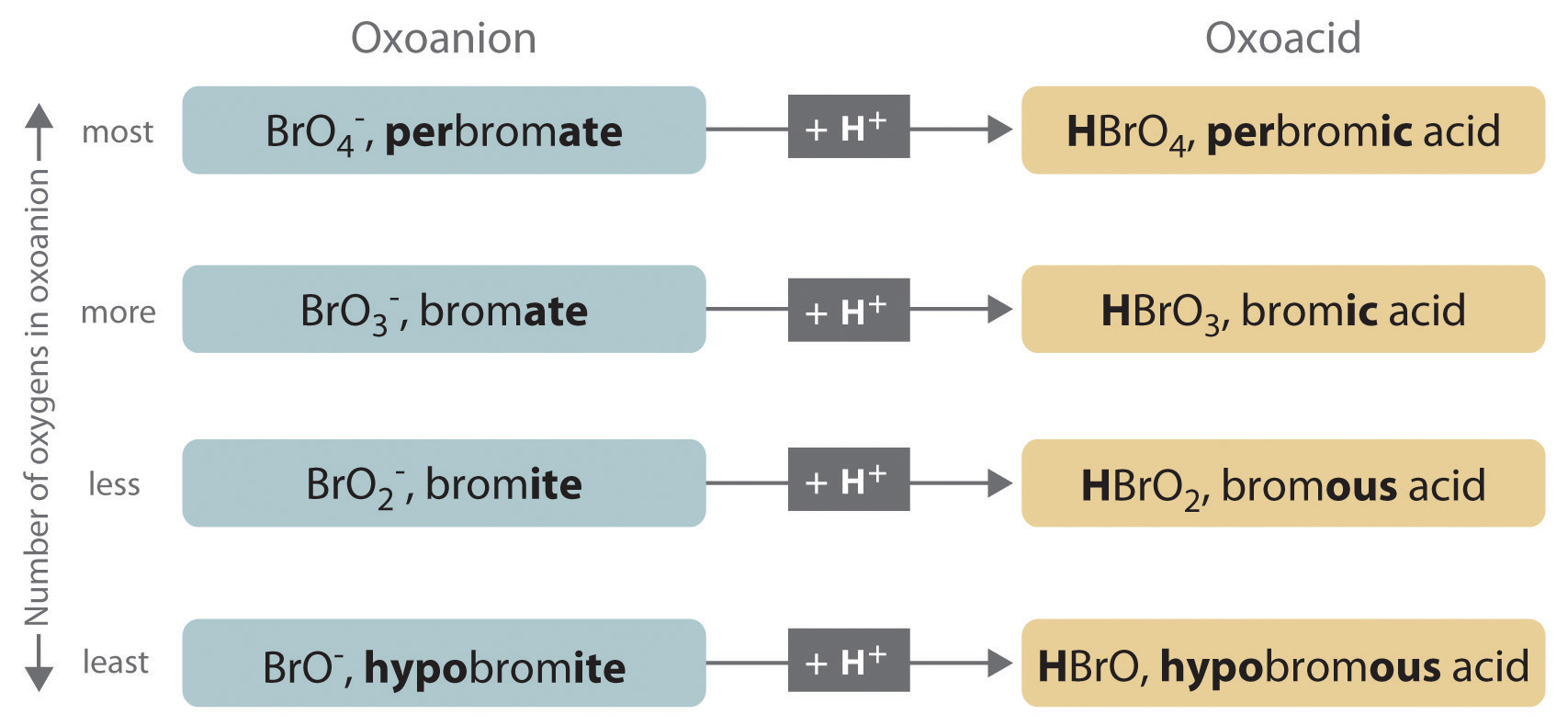Table 2.9 Some Common Oxoacids

Formula Name
HNO2 nitrous acid
HNO3 nitric acid
H2SO3 sulfurous acid
H2SO4 sulfuric acid
H3PO4 phosphoric acid
H2CO3 carbonic acid
HClO hypochlorous acid
HClO2 chlorous acid
HClO3 chloric acid
HClO4 perchloric acid

### Example 11

Name and give the formula for each acid.

1. the acid formed by adding a proton to the hypobromite ion (OBr)
2. the acid formed by adding two protons to the selenate ion (SeO42−)

Given: anion

Strategy:

Refer to Table 2.8 "Some Common Acids That Do Not Contain Oxygen" and Table 2.9 "Some Common Oxoacids" to find the name of the acid. If the acid is not listed, use the guidelines given previously.

Solution:

Neither species is listed in Table 2.8 "Some Common Acids That Do Not Contain Oxygen" or Table 2.9 "Some Common Oxoacids", so we must use the information given previously to derive the name of the acid from the name of the polyatomic anion.

1. The anion name, hypobromite, ends in -ite, so the name of the parent acid ends in -ous. The acid is therefore hypobromous acid (HOBr).
2. Selenate ends in -ate, so the name of the parent acid ends in -ic. The acid is therefore selenic acid (H2SeO4).

Exercise

Name and give the formula for each acid.

1. the acid formed by adding a proton to the perbromate ion (BrO4)
2. the acid formed by adding three protons to the arsenite ion (AsO33−)

1. perbromic acid; HBrO4
2. arsenous acid; H3AsO3

Many organic compounds contain the carbonyl groupA carbon atom double-bonded to an oxygen atom. It is a characteristic feature of many organic compounds, including carboxylic acids., in which there is a carbon–oxygen double bond. In carboxylic acidsAn organic compound that contains an −OH group covalently bonded to the carbon atom of a carbonyl group. The general formula of a carboxylic acid is $RCO2H$. In water a carboxylic acid dissociates to produce an acidic solution., an –OH group is covalently bonded to the carbon atom of the carbonyl group. Their general formula is RCO2H, sometimes written as RCOOH:where R can be an alkyl group, an aryl group, or a hydrogen atom. The simplest example, HCO2H, is formic acid, so called because it is found in the secretions of stinging ants (from the Latin formica, meaning “ant”). Another example is acetic acid (CH3CO2H), which is found in vinegar. Like many acids, carboxylic acids tend to have sharp odors. For example, butyric acid (CH3CH2CH2CO2H), is responsible for the smell of rancid butter, and the characteristic odor of sour milk and vomit is due to lactic acid [CH3CH(OH)CO2H]. Some common carboxylic acids are shown in Figure 2.21 "Some Common Carboxylic Acids".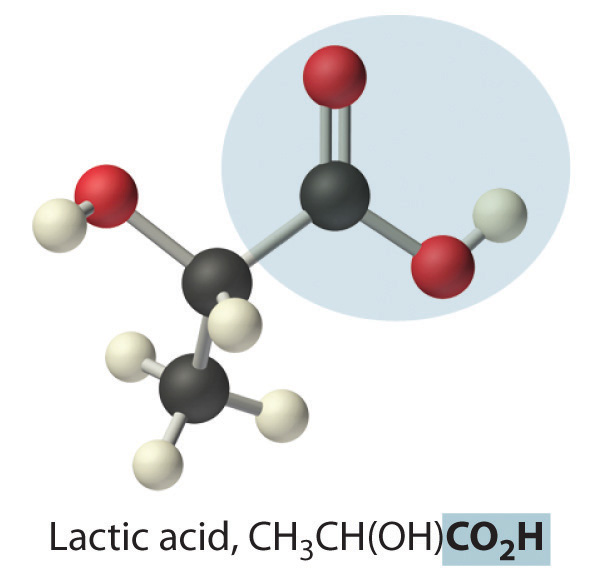Figure 2.21 Some Common Carboxylic Acids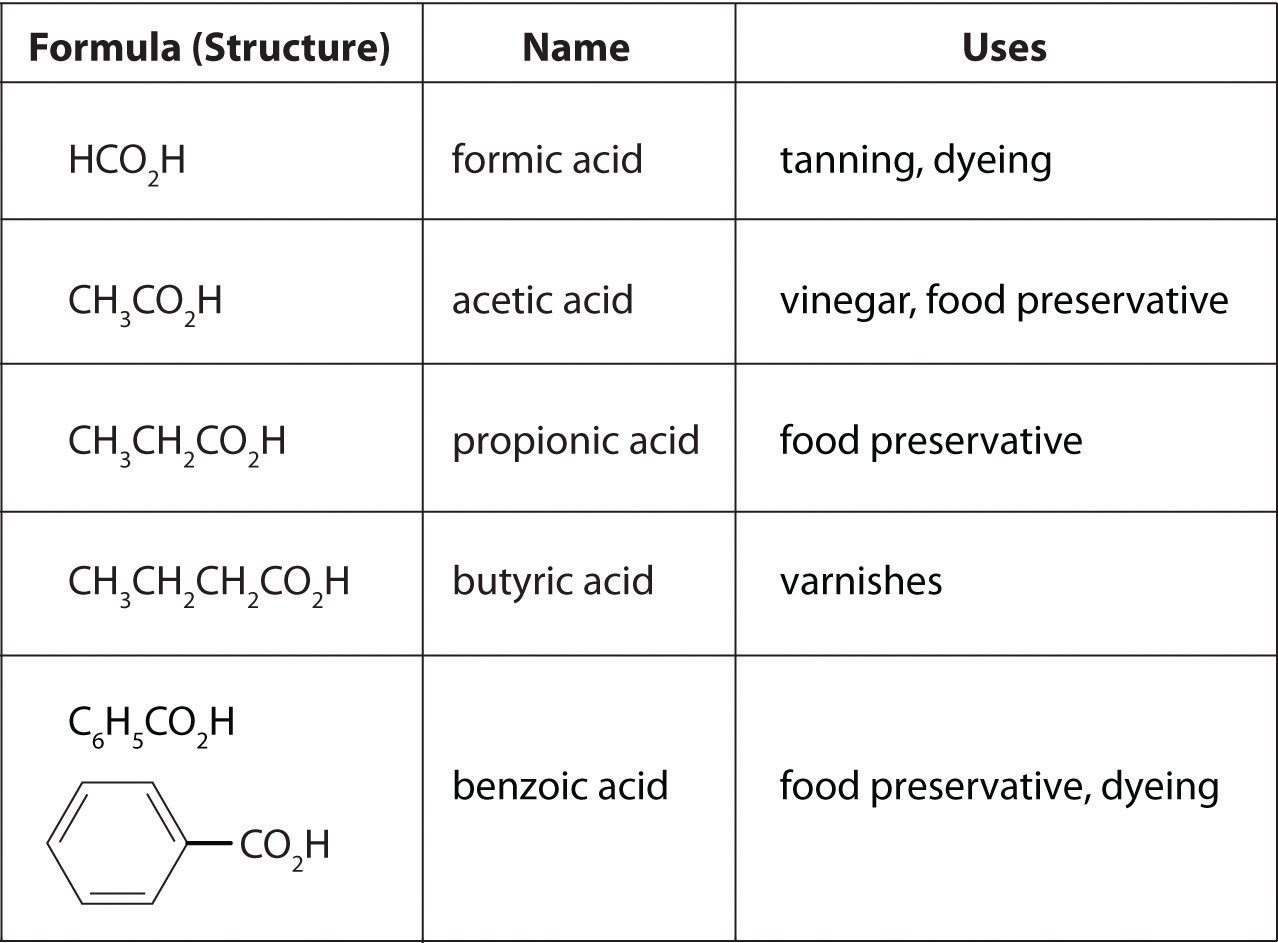Although carboxylic acids are covalent compounds, when they dissolve in water, they dissociate to produce H+ ions (just like any other acid) and RCO2 ions. Note that only the hydrogen attached to the oxygen atom of the CO2 group dissociates to form an H+ ion. In contrast, the hydrogen atom attached to the oxygen atom of an alcohol does not dissociate to form an H+ ion when an alcohol is dissolved in water. The reasons for the difference in behavior between carboxylic acids and alcohols will be discussed in Chapter 8 "Ionic versus Covalent Bonding".

### Note the Pattern

Only the hydrogen attached to the oxygen atom of the CO2 group dissociates to form an H+ ion.

## Bases

We will present more comprehensive definitions of bases in later chapters, but virtually every base you encounter in the meantime will be an ionic compound, such as sodium hydroxide (NaOH) and barium hydroxide [Ba(OH)2], that contain the hydroxide ion and a metal cation. These have the general formula M(OH)n. It is important to recognize that alcohols, with the general formula ROH, are covalent compounds, not ionic compounds; consequently, they do not dissociate in water to form a basic solution (containing OH ions). When a base reacts with any of the acids we have discussed, it accepts a proton (H+). For example, the hydroxide ion (OH) accepts a proton to form H2O. Thus bases are also referred to as proton acceptors.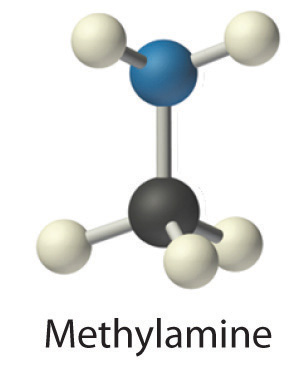Concentrated aqueous solutions of ammonia (NH3) contain significant amounts of the hydroxide ion, even though the dissolved substance is not primarily ammonium hydroxide (NH4OH) as is often stated on the label. Thus aqueous ammonia solution is also a common base. Replacing a hydrogen atom of NH3 with an alkyl group results in an amineAn organic compound that has the general formula $RNH2$, where R is an alkyl group. Amines, like ammonia, are bases. (RNH2), which is also a base. Amines have pungent odors—for example, methylamine (CH3NH2) is one of the compounds responsible for the foul odor associated with spoiled fish. The physiological importance of amines is suggested in the word vitamin, which is derived from the phrase vital amines. The word was coined to describe dietary substances that were effective at preventing scurvy, rickets, and other diseases because these substances were assumed to be amines. Subsequently, some vitamins have indeed been confirmed to be amines.

### Note the Pattern

Metal hydroxides (MOH) yield OH ions and are bases, alcohols (ROH) do not yield OH or H+ ions and are neutral, and carboxylic acids (RCO2H) yield H+ ions and are acids.

### Summary

Common acids and the polyatomic anions derived from them have their own names and rules for nomenclature. The nomenclature of acids differentiates between oxoacids, in which the H+ ion is attached to an oxygen atom of a polyatomic ion, and acids in which the H+ ion is attached to another element. Carboxylic acids are an important class of organic acids. Ammonia is an important base, as are its organic derivatives, the amines.

### Key Takeaway

• Common acids and polyatomic anions derived from them have their own names and rules for nomenclature.

### Conceptual Problems

1. Name each acid.

1. HCl
2. HBrO3
3. HNO3
4. H2SO4
5. HIO3
2. Name each acid.

1. HBr
2. H2SO3
3. HClO3
4. HCN
5. H3PO4
3. Name the aqueous acid that corresponds to each gaseous species.

1. hydrogen bromide
2. hydrogen cyanide
3. hydrogen iodide
4. For each structural formula, write the condensed formula and the name of the compound.

1.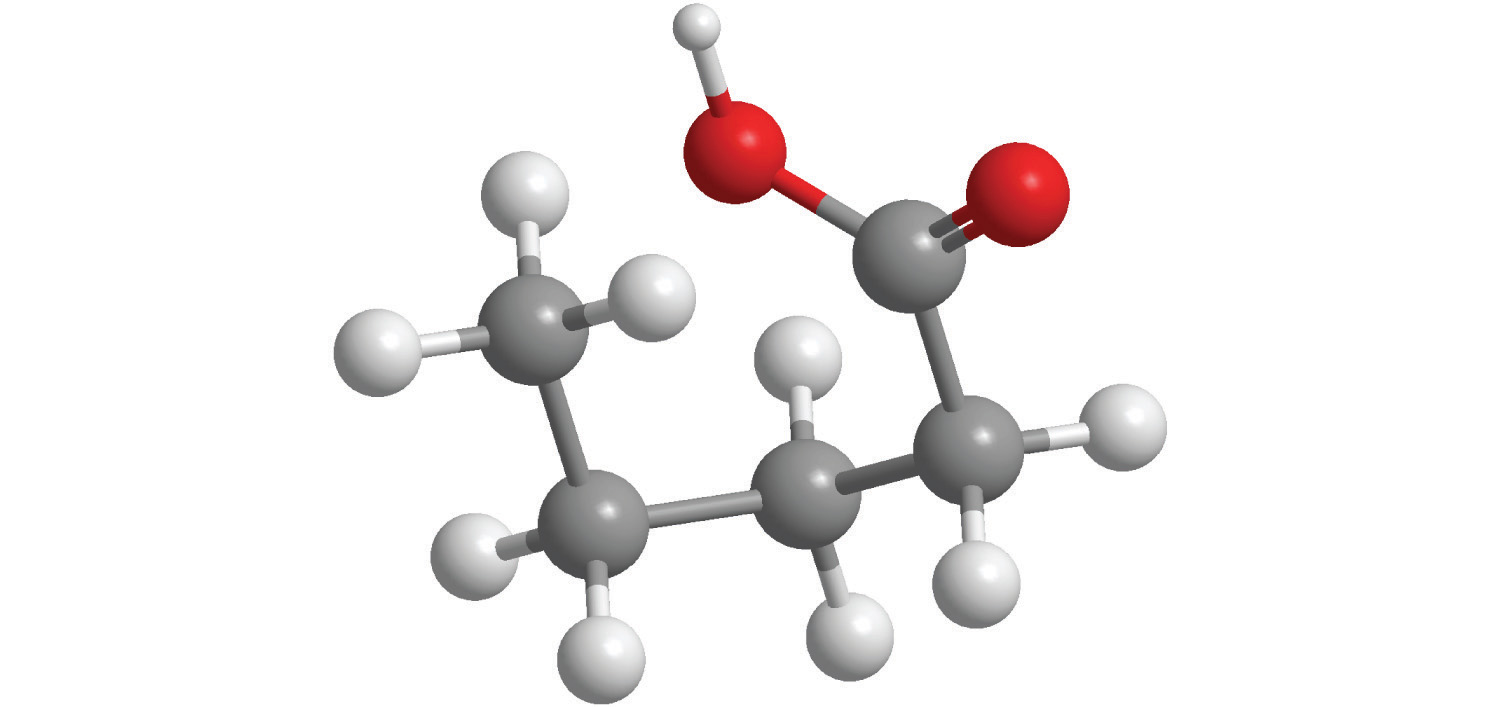2.5. For each structural formula, write the condensed formula and the name of the compound.

1.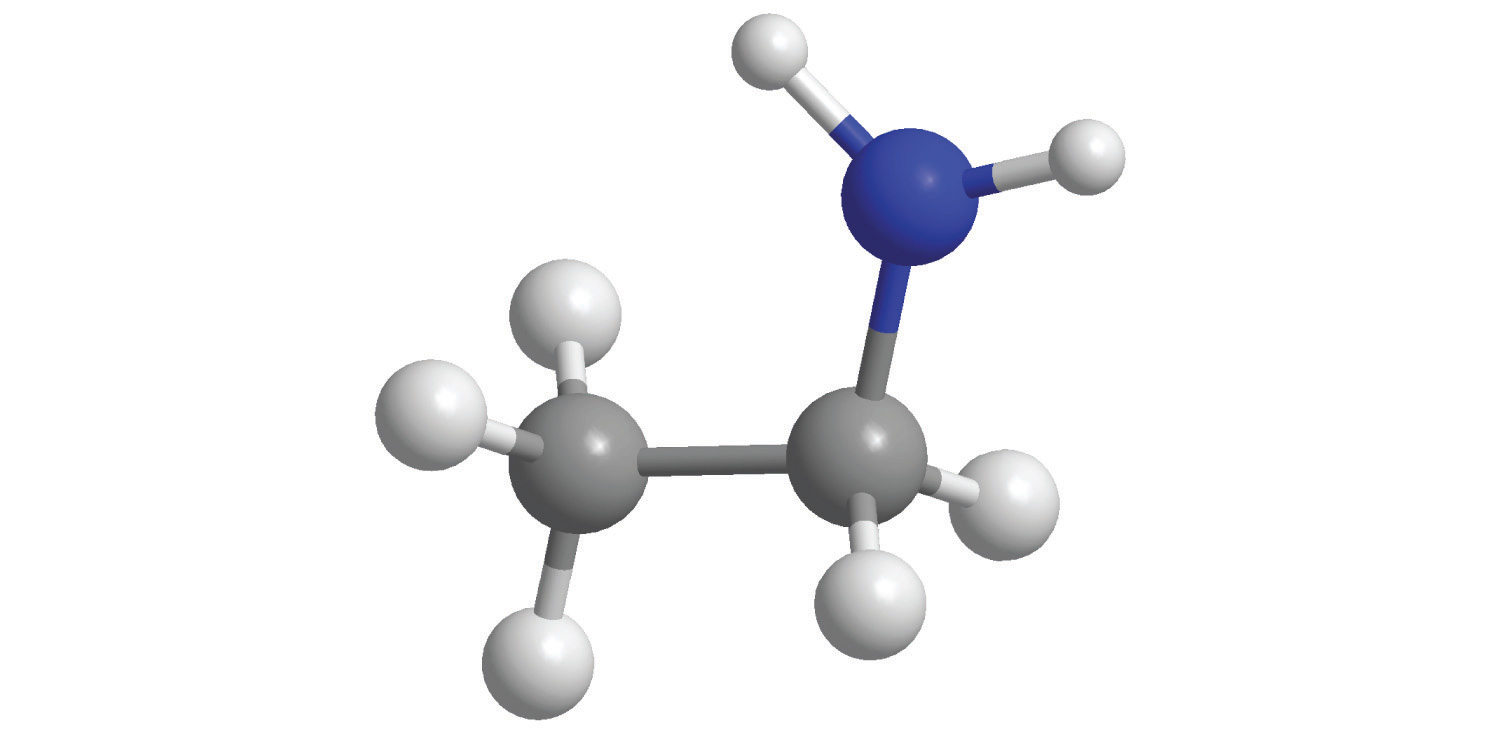2.6. When each compound is added to water, is the resulting solution acidic, neutral, or basic?

1. CH3CH2OH
2. Mg(OH)2
3. C6H5CO2H
4. LiOH
5. C3H7CO2H
6. H2SO4
7. Draw the structure of the simplest example of each type of compound.

1. alkane
2. alkene
3. alkyne
4. aromatic hydrocarbon
5. alcohol
6. carboxylic acid
7. amine
8. cycloalkane
8. Identify the class of organic compound represented by each compound.

1.2. CH3CH2OH
3. HC≡CH
4.5. C3H7NH2
6. CH3CH=CHCH2CH3
7.8.9. Identify the class of organic compound represented by each compound.

1.2.3.4.5.6. CH3C≡CH
7.8.### Numerical Problems

1. Write the formula for each compound.

1. hypochlorous acid
2. perbromic acid
3. hydrobromic acid
4. sulfurous acid
5. sodium perbromate
2. Write the formula for each compound.

1. hydroiodic acid
2. hydrogen sulfide
3. phosphorous acid
4. perchloric acid
5. calcium hypobromite
3. Name each compound.

1. HBr
2. H2SO3
3. HCN
4. HClO4
5. NaHSO4
4. Name each compound.

1. H2SO4
2. HNO2
3. K2HPO4
4. H3PO3
5. Ca(H2PO4)2·H2O